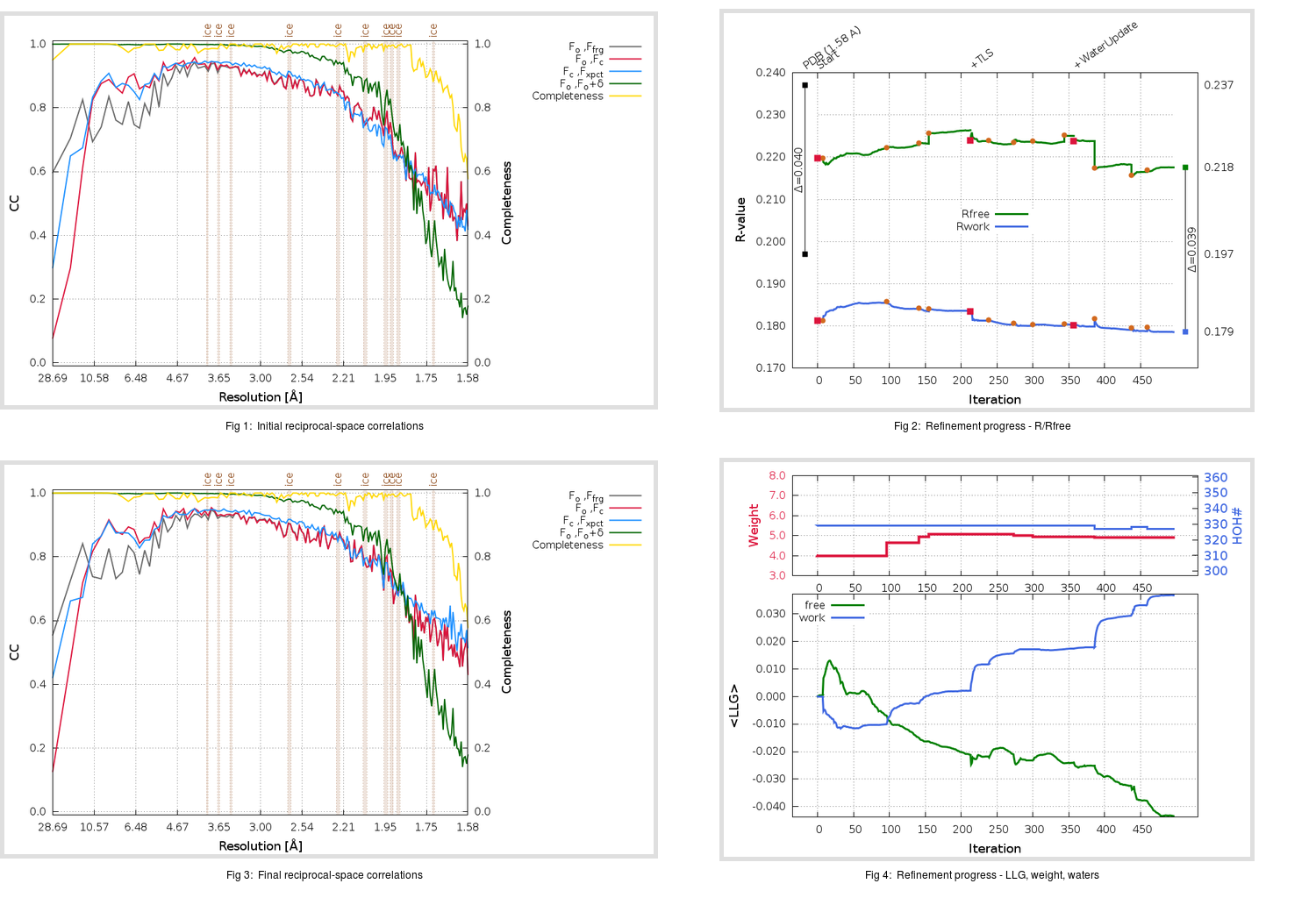Content:

## Deposited

` `
 Date deposited Date data collection Resolution R, Rfree 20200315 20200305 1.58 0.1950 0.2370

Molprobity (CCP4 7.0 version) summary:

```Ramachandran outliers =   0.33 %
favored =  97.68 %
Rotamer outliers      =   1.52 %
C-beta deviations     =     0
Clashscore            =   4.22
RMS(bonds)            =   0.0128
RMS(angles)           =   1.81
MolProbity score      =   1.41
Resolution            =   1.58
R-work                =   0.1950
R-free                =   0.2370
```

```Number of waters      =   329

<B> (all atoms) =   24.95 ( sd =    8.59 ) for       2730 non-hydrogen atoms
<B>   (protein) =   23.55 ( sd =    7.46 ) for       2370 non-hydrogen atoms
<B>     (water) =   34.25 ( sd =    9.66 ) for        329 non-hydrogen atoms
<B>    (others) =   32.26 ( sd =   11.12 ) for         31 non-hydrogen atoms

B min/max       (all non-hydrogen atoms) =   13.91 /   67.71
B min/max   (protein non-hydrogen atoms) =   13.91 /   65.90
B min/max     (water non-hydrogen atoms) =   15.03 /   67.71
B min/max     (other non-hydrogen atoms) =   24.36 /   46.47
```

## BUSTER (re-)refinement

` `

Molprobity (CCP4 7.0 version) summary:

```Ramachandran outliers =   0.33 %
favored =  98.34 %
Rotamer outliers      =   1.52 %
C-beta deviations     =     0
Clashscore            =   1.69
RMS(bonds)            =   0.0114
RMS(angles)           =   1.54
MolProbity score      =   1.06
Resolution            =   1.58
R-work                =   0.1786
R-free                =   0.2177
```

```Number of waters      =   327

<B> (all atoms) =   27.09 ( sd =    8.28 ) for       2728 non-hydrogen atoms
<B>   (protein) =   25.51 ( sd =    6.58 ) for       2370 non-hydrogen atoms
<B>     (water) =   37.72 ( sd =   10.26 ) for        327 non-hydrogen atoms
<B>    (others) =   34.47 ( sd =   12.68 ) for         31 non-hydrogen atoms

B min/max       (all non-hydrogen atoms) =   13.82 /   66.23
B min/max   (protein non-hydrogen atoms) =   13.82 /   58.89
B min/max     (water non-hydrogen atoms) =   15.86 /   66.23
B min/max     (other non-hydrogen atoms) =   27.95 /   51.97
```

Refinement progression:Results:

` `
 File Remark 5RFT_aB_refine.01_03_refine.pdb.gz exact refinement commands are in header 5RFT_aB_refine.01_03_refine.mtz.gz including original deposited data and several re-refinement map coefficients 5RFT_aB_refine.01_03_BUSTER_model.cif.gz including any non-standard compound restraints 5RFT_aB_refine.01_03_BUSTER_refln.cif.gz